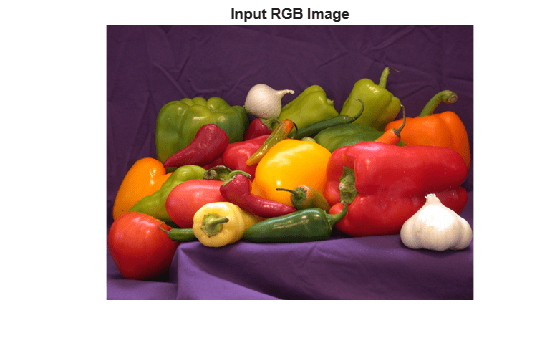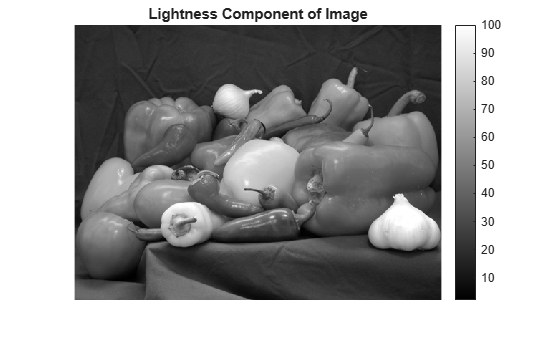# rgb2lightness

Convert RGB color values to lightness values

## Syntax

``lightness = rgb2lightness(rgb)``

## Description

example

````lightness = rgb2lightness(rgb)` converts RGB color values to lightness values, excluding the color components. `lightness` is same as the L* component in the CIE 1976 L*a*b* color space.```

## Examples

collapse all

Read RGB image into the workspace.

`rgb = imread('peppers.png');`

Convert the RGB color values to lightness component, excluding the color information.

`lightness = rgb2lightness(rgb);`

Display the RGB image and the derived lightness component of image.

```figure imshow(rgb) title('Input RGB Image')``````figure imshow(lightness,[]) title('Lightness Component of Image') colorbar```## Input Arguments

collapse all

RGB color values, specified as an m-by-n-by-3 image array. The input `rgb` must be in sRGB color space with a reference white point of D65.

Data Types: `single` | `double` | `uint8` | `uint16`

## Output Arguments

collapse all

Converted lightness values, returned as an m-by-n image array. If the input data type is `double`, the output data type is `double`. Otherwise, the output data type is `single`.

Data Types: `single` | `double`

## Version History

Introduced in R2019a

expand all